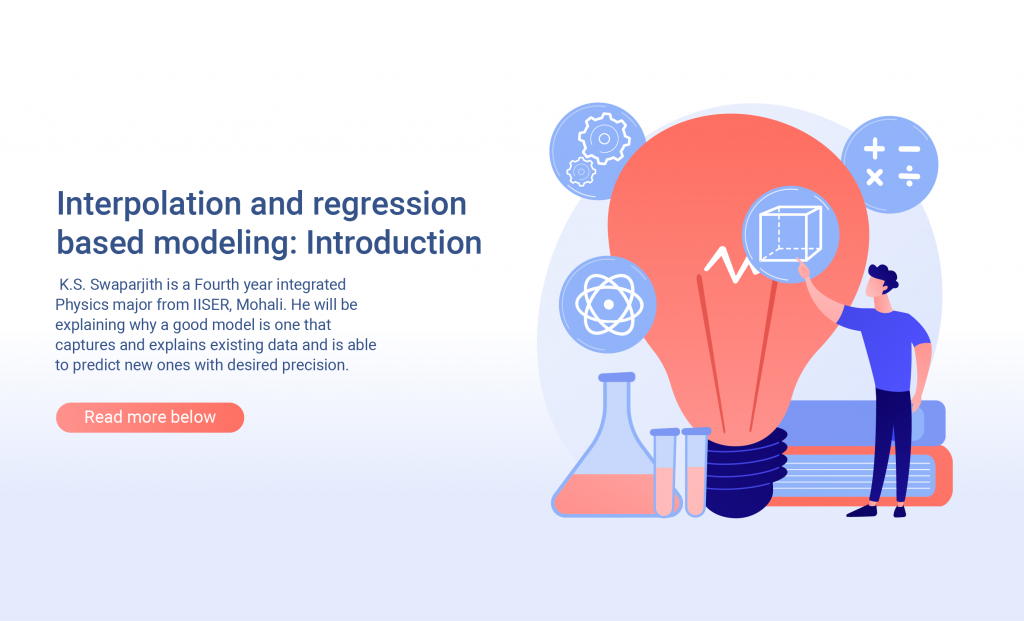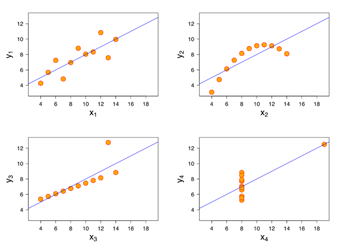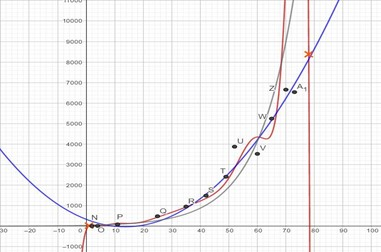## An introduction to interpolation and regression based modeling

K.S. Swaparjith is a Fourth year Integrated Physics major from IISER, Mohali. He will be explaining why a good model is one that captures and explains existing data and is able to predict new ones with desired precision.Introduction

The COVID-19 pandemic, amidst its horrors of damage to lives and economies and a complete reshaping of our social interactions, has brought quite a few technical words into our daily lives. Words like modelling, simulation, growth curve, the exponential curve, predictions, projections, curve flattening and so on are being used constantly to usher in new pandemic management policies.

It is in this context that it becomes purposeful to address some of these words so that they can be accessed without much loss of mathematical content and to provide a peek into the essentials of building a model to understand a novel situation. Building a model for an observed event basically implies identifying a mathematical function that best fits the observation and enables meaningful predictions. Essentially this article aims to introduce model building without slipping into a crash course format on statistics.

By the end of this the reader will be familiar with terms like regression, interpolating polynomial and extrapolation. The reader will also be exposed to some of the intricacies and the difficulties that are present while constructing a model.  For this purpose, the relevant analysis and presentation of images are constructed on GeoGebra using simple inbuilt tools.

Preliminaries

Consider either a natural phenomenon, be it growth of a bacterial culture or spreading of a pandemic, or planned experiments to determine variation of resistance of a material or even a social event such as poll results that require to be explained. Firstly, it is imperative to identify an independent variable in such a way that the observation does not influence it. For instance, in the case of bacterial growth it could be time or the number of oxygen cylinders needed with respect to the number of Covid-positive cases.

In the case of planned experiments, after identifying the suitable independent variable(s) the experiments are performed to get values of the dependent variable corresponding to various settings of the independent variable. Use of the best available technology over a wide range with fine step size mandates good quality data collection. Sometimes the phenomenon may be rare (e.g. LIGO, Higgs Boson etc.) thus limiting the number of data. Once sufficient data are collected, a relationship in a functional form can be achieved from curve fitting or from modelling. Against this backdrop, let us now try something interesting.

Methods of modelling

We start with a set of data (points A to G) that have been generated by performing an experiment. Table. 1 presents the observed data and Figure 1 is a scatter plot of the same. Let the various values of the independent variable be denoted by xi and the dependent variable yi. We attempt to model the observed information using a function of the form f(x,bj) where bi are parameters of the function that  needs to be determined. In this introductory article we restrict to making models out of polynomial functions.

 Label xi yi A 3.14 3.14 B 9.42 9.42 C 12.18 11.18 D 13.05 13.52 E 16.06 15.72 F 18.85 18.85 G 22.56 22.02

Table 1: SEQ Table \* ARABIC 1 the original experimental data points

We impose a condition that the model function f(x,bi) should either pass through all the data points  of the experiment that is yi=f(xi,bj) for all i or should be constrained to pass  such  that its deviation, (yi-f(xi,bj))2 from the experimental data is the minimum. We must note that there are other ways to define deviation. We have used the least square deviation. One could also use the absolute value of the difference. Having made the choice of polynomial function, the task is to determine the parameters. Two mathematical methods, (a) interpolation and (b) regression emerge in this process. In the interpolation method, we demand that the polynomial passes through all the points. In this approach, when there are n data points from the experiment, we can fit a polynomial of order n-1 with n parameters (b0 to bn-1):

y=bn-1xn-1 + bn-2xn-2 + bn-3xn-3 +… b1x1 +b0

We now have n such equations. Indeed, we can solve a system of n linear equations with n variables either manually or by using a computer algorithm and determine the parameters. For this the software “GeoGebra” has been used here. Thus, we obtain the interpolating polynomial with which values of y for all values of x within the range of interpolation (the point A and point G) can be predicted.  Figure 2 shows that the polynomial does go through all the data points.

Moving over to the second approach, regression analysis, we can fit polynomials of lesser order. Here we have an over-determined system of n equations with m parameters where n>m. However, we have the other constraint of minimizing deviation between the experimental and fitted data points, i.e (yi-f(xi,bj))2.

The fit obtained from both regression and interpolation is presented in Figure 3 whereas the individual regression fits are presented in Figures 4 to 8.

Predictability within the range

We started off with an experimental data set (points A to G) and constructed 6 polynomial models. To identify the model that best predicts experimental values we repeat the experiment at a new set of points in the range A to G (points H to N). Simultaneously we make predictions using the 6 different models and evaluate the deviation from the experiment.

Figures 9 and 10 show the extent of deviation from the linear and sixth order polynomial predictions with the actual experimental values. A detailed list of such deviations for the other orders of polynomials is presented in Table 2.

Notice that in the case of the interpolating polynomial, there is very little deviation in some range but very large deviation near the extremities. The linear fit has about the same deviation throughout. That is the interpolating polynomial has a region of very little deviation (or high predictability).

To add further strength to the above observation, when the experiment is repeated using the six models, the deviations are the same as those calculated previously.  The results of this effort are presented in Figure 11 and Table 3.

Again, excluding the extremities, the interpolating polynomial has the least deviation. This implies that one can predict the outcomes of a phenomenon with very little error for unknown values of the independent variable in this range. We can conclude that for the 7 experimental data points, the 6th order polynomial is the best model in the specified range.

Let us now ask the following questions: what about predictability beyond the initial range? Are we anywhere close to reality?

The answer to the first question is no. We cannot make predictions using an interpolation polynomial for regions beyond the initial observations. Predicting beyond the range, namely extrapolation, requires extensive experimentation and establishing a tight relation.  Extrapolation with limited data and analysis is sure to be erroneous. This also means that the more data points we have the better curve fit we get.

The second question dominates a significant part of the entire field of modeling. To know if we have a model that represents reality, we would need more data. The data, used along with “educated guesses” and intuition is really the only way out. The guesses can come from observations of similar phenomena or based on other analogous experiences. Things become further complicated when there are more parameters to consider. For instance, consider the 21 data points that we have experimentally generated so far and attempt to improve the model. Figure 12 shows the scatter plot.

Modelling this with a polynomial of order 20 as before is shown in Figure 13. We also observe that the data points show wiggle and periodicity and would like to examine if this visual input can be included in modelling. Recall that the linear fit had almost a constant average deviation irrespective of the number of points, unlike the interpolating polynomial with large errors at the extremities. So, one can posit that there might be a linear term that is causing the rise. We know that trigonometric functions can be invoked for wiggles and they are also periodic. Hence a combination of these two functions of the form mx+c+a.sin(b.x+d), with five parameters can be tried.

Figure 14: (a, b) modelling with sin(x)+x and the 6th order and 20th order interpolations

The result of this analysis along with those of the earlier interpolation polynomial approach presented in Figure 14(a, b) reveals the potential of educated guess though one has started with a rudimentary polynomial. In general, an awareness of other functional forms including complex Legendre, Chebyshev polynomials and Fourier series will aid the guess work in the pursuit of revealing the reality.

At this juncture it is interesting to familiarize with the famous Anscombe’s quartet (Figure 15).  A data set generated by Francis Anscombe shows that analyzing data just as numbers is not enough. Though the models share the same statistical properties, such as mean and standard deviation with the data, they fail to capture the nature of the data.Figure 15: The Anscombe quartet. The orange dots show the actual data. The line shows the best model that has the same statistical properties. (image source: Wikipedia)

On the present pandemic

Let us try to apply the tools from the above study on actual Covid-19 data from India.  Data points scattered over the previous months have been picked to see how the various fits predict the intermediate data. Figure 16 shows daily increase in cases in India from the date from 15th of March (Data Courtesy: Govt. source through COVID-19 tracker).

 Label x (day) y (daily increase) A 1 26 B 5 22 C 9 108 D 13 140 E 17 146 F 26 485 G 33 826 H 40 1229 I 47 1823 J 51 2573 K 58 4213 L 66 4970 M 78 8380

Table 4: Raw data

Figures 17 and 18 show the interpolating polynomial fit and the lower order regression fits for the Covid-19 data.

Yet again we repeat our analysis by observing the deviations between model predictions and actual data from intermediate days (Table 5 and Figure 19).  We have also included the growth curve, a function of the form a.bxFigure 19: Image showing the scatter plot of actual increase vs the various models computed from the initial data

Both the interpolating polynomial and the growth curve show vast average deviation.  It is a quadratic fit that provides the least deviation in this range. However, we cannot conclude the pandemic data to be a quadratic polynomial. As seen from the example with the Anscombe data, one cannot easily conclude that minimum deviation implies the right model. There is a drastic difference that can be seen (in Table 5) between the deviation of the special points and that of the entire data. While it can also be seen that the difference between the quadratic and the two other polynomials is quite low. These two indicate that a few deviant data points can drastically change the best fit and thus a comprehensive model would require more data and parameters.

Conclusion

A multiparameter phenomenon like a pandemic is complex. It requires much more comprehensive data collection, careful inspection and understanding complex interdependencies of various factors. The study of modeling and prediction is vast and still growing. This article has attempted to shed some light on the complex and involved nature of the process of modeling and its crucial dependence on experimental data. This also shows that reliance on such simple ideas of curve fitting is necessary but not sufficient to generate a comprehensive and predictable model. Though not a key aspect of this article in real life, extrapolation becomes the primary interest. While identifying important parameters and recognizing patterns is essential to build a consistent and predictable model one has to ultimately be sensitive to new data and experiments as that alone is reality.

A good model ultimately is one that captures and explains existing data and is able to predict new ones with desired precision.

### K.S. Swaparjith

Fourth year integrated Physics major
IISER, Mohali